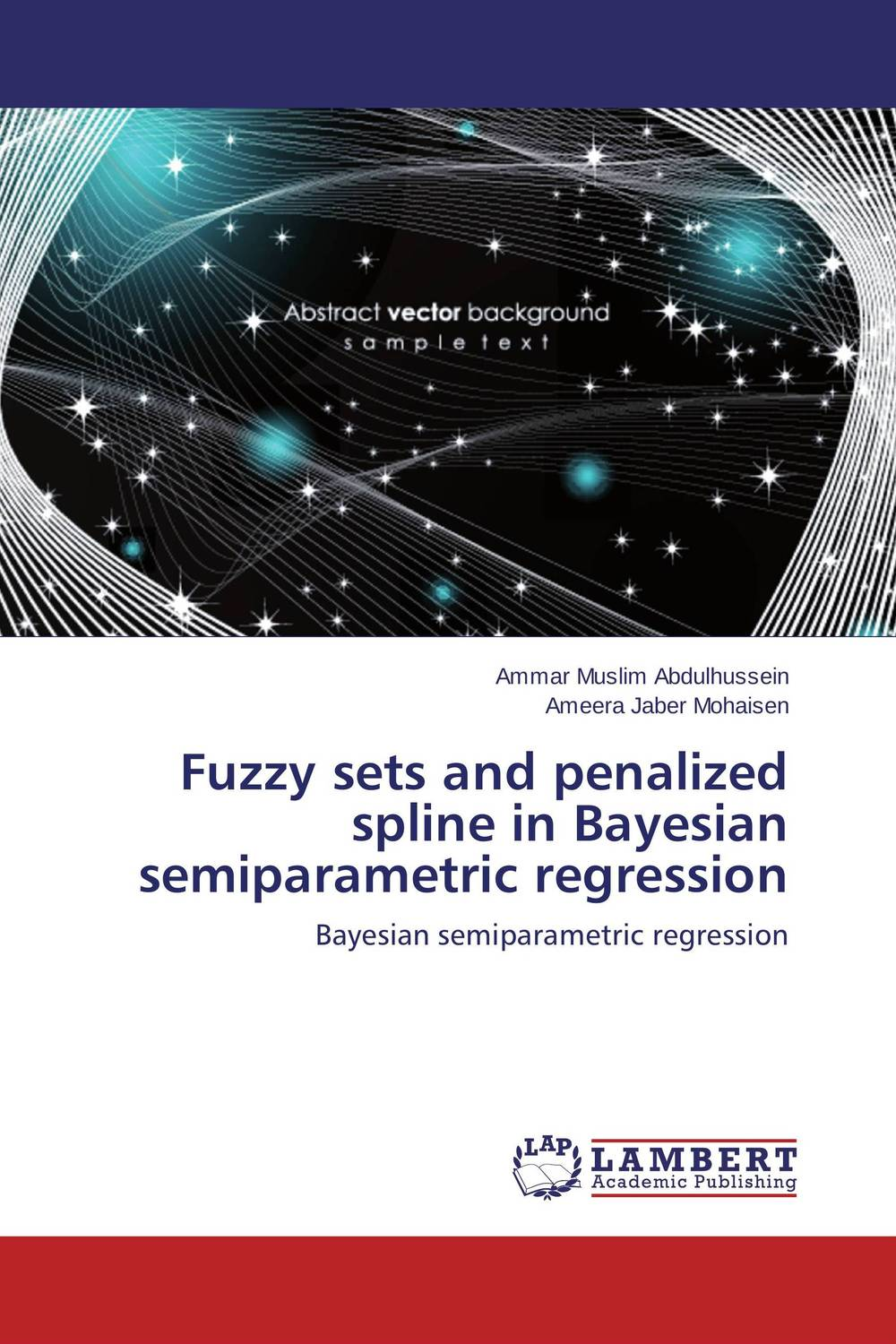# Fuzzy sets and penalized spline in Bayesian semiparametric regressionГод: 2014
Автор: Ammar Muslim Abdulhussein and Ameera Jaber Mohaisen
Издательство: LAP Lambert Academic Publishing
Описание:
We consider semiparametric regression model where the mean function of this model has two parts, the parametric is assumed to be linear function of p-dimensional covariates and nonparametric is assumed to be a smooth penalized spline. By using a convenient connection between penalized splines and mixed models, we can represent semiparametric regression model as a mixed model. Bayesian approach is employed to make inferences on the resulting mixed model coefficients, and we prove some theorems about posterior. We also investigate the large sample property of the Bayes factor for testing the polynomial component of spline model against the fully spline semiparametric alternative model, as well as Bayesian approach to semiparametric regression model which is described by using fuzzy sets and membership functions. The membership functions are interpreted as likelihood functions for the model, furthermore, we prove some theorems about posterior and Bayes factor in this case.
This entry was posted in Без рубрики on by .